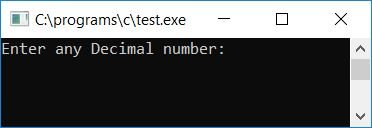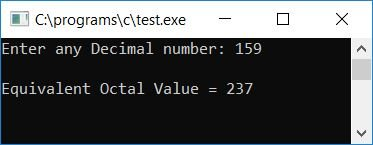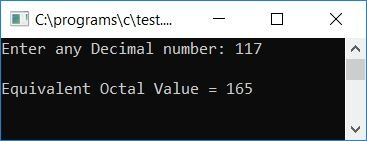# C Program to Convert Decimal to Octal

In this article, we will learn how to create a program in C that converts any given decimal number (given by the user at run-time) to its equivalent octal number. At last, we have also created a user-defined function program that does the same job.

Before going through the program, if you are not aware of

• Decimal Number
• Octal Number
• Decimal to Octal Conversion

then refer to the step-by-step process for decimal to octal conversion. Now let's move on to the program.

## Decimal to octal in C

To convert a decimal number to an octal number in C programming, you have to ask the user to enter the decimal number, convert it to an octal number, and then display the equivalent value in octal as an output, as shown here in the program given below:

```#include<stdio.h>
#include<conio.h>
int main()
{
int decnum, octnum, i=0;
printf("Enter any Decimal number: ");
scanf("%d", &decnum);
while(decnum != 0)
{
octnum[i] = decnum%8;
i++;
decnum = decnum/8;
}
printf("\nEquivalent Octal Value = ");
for(i=(i-1); i>=0; i--)
printf("%d", octnum[i]);
getch();
return 0;
}```

As the above program was written in the Code::Blocks IDE, here is the sample run after a successful build and run. This is the first snapshot of the sample run:Now supply any decimal number, say 159, and press the ENTER key to see its equivalent octal value as shown in the second snapshot of the sample run given here:#### Program Explained

• Receive any decimal number from the user at program runtime as an input.
• Create a while loop that runs until the value of the entered decimal number is not equal to zero (0). In other words, the while loop runs until the value of decnum (the given decimal number) becomes 0.
• Let's suppose that the user has entered 159 as input. Therefore, at the first run of the while loop, the condition decnum!=0 or 159!=0 evaluates to true, and therefore program flow goes inside the loop.
• and decnum%8 or 159%8 (while dividing the number 159 by 8, we will get a remainder of 7), or 7 gets initialized to octnum[i] or octnum (as i holds 0 as its initial value).
• Now, the value of i gets incremented and becomes 1. And then decnum/8 or 159/8 or 19 gets initialized to decnum.
• Now the program flow goes back to the while loop and checks the condition, which is that decnum!=0 or 19!=0 evaluates to true. Therefore, the program flow again goes inside the loop, and decnum%8 or 19%8 or 3 gets initialized to octnum[i] or octnum.
• The value of i gets incremented and becomes 2. And then decnum/8 or 19/8 or 2 gets initialized to decnum. The program flow goes back to the while loop and evaluates the condition.
• That is, either decnum!=0 or 2!=0 is true, so the program goes back into the loop.
• And decnum%8 or 2%8 (speaking only of whole numbers, 2 cannot be divided by 8, so 2 is the remainder in this case) or 2 is initialized to octnum[i] or octnum.
• The value of i gets incremented and becomes 3. And decnum/8, 2/8, or 0 is set to decnum.
• The program flow again goes back to the while loop and evaluates the condition, which is decnum! = 0 or 0! =0 evaluates to false, therefore program flow does not go inside the loop (that is, now the program flow exits the loop).
• We have now stored all of the equivalent octal digits of the given decimal number in an array called octnum [].
• We have to create a for loop that will be initialized with one less than the current value of i. We incremented the value of i in the previous run of the while loop. However, as decnum approaches zero, the program flow exits the loop; however, i retains its value as one greater than the required value; thus, we must delete or skip the last index or number.
• We have to print the octal digits one by one in reverse order. That is, the loop runs from i-1 to a value greater than or equal to 0.
• Inside the loop, print the octal digit one by one.
• In this way, we have printed the equivalent octal value of the given decimal number to the user at run-time.

### Decimal to Octal in C without a Modulus Operator

Now, let's make the same program that converts any given decimal number into its equivalent octal value without using any modulus operators, as shown in the code below.

```#include<stdio.h>
#include<conio.h>
int main()
{
int decnum, octnum, i=0, temp, chck, rem;
printf("Enter any Decimal number: ");
scanf("%d", &decnum);
while(decnum!=0)
{
temp = decnum/8;
chck = temp*8;
rem = decnum - chck;
octnum[i] = rem;
i++;
decnum = temp;
}
printf("\nEquivalent Octal Value = ");
for(i=i-1; i>=0; i--)
printf("%d", octnum[i]);
getch();
return 0;
}```

Here is the final snapshot of the sample run:#### Program Explained

• As we all know, the modulus operator (%) only finds the remainder when dividing any number by another number.
• So, we have to find the number left over when we divide the number in the "decnum" variable by 8.
• So, we just divided the number by 8 and put the result into a variable we'll call "temp."
• And then we have again multiplied the same number, temp, with 8 and initialized another variable, say "chk."
• Subtract and assign the value of "decnum - chck" (the original number) to a variable called rem.
• For example, let's suppose the user has supplied 159 as input.
• Therefore, at the first run of the while loop, decnum/8 or 159/8 or 19 gets initialized to temp.
• And temp*8 or 19*8 or 152 is set to check.
• Then, decnum-chk or 159-152 or 7 gets initialized to rem.
• If you replace the three line codes with rem = decnum%8, you will get the same result, i.e., 7 will be initialized to rem here.

Therefore, we have successfully replaced the modulus operator and created the program for the same purpose but without using any modulus operators as shown in the above program.

### Decimal to Octal in C using a User-Defined Function

Now let's create a function-driven program for the same purpose. The question is, "Write a program in C that converts a decimal number to an octal number using a user-defined function." The answer to this question is:

```#include<stdio.h>
#include<conio.h>
void DecToOct(int dec);
int octnum;
static int i;
int main()
{
int decnum;
printf("Enter any Decimal number: ");
scanf("%d", &decnum);
DecToOct(decnum);
printf("\nEquivalent Octal Value = ");
for(i=(i-1); i>=0; i--)
printf("%d", octnum[i]);
getch();
}
void DecToOct(int dec)
{
while(dec != 0)
{
octnum[i] = dec%8;
i++;
dec = dec/8;
}
}```

Here is the final snapshot of the sample run of the above program:Here we have declared the variable i as a static variable. because a static variable remembers its previous value. And we have not initialized the value of i with 0, because a static variable holds 0 as its initial value. That is, if you do not assign any value to any static variable, 0 is assigned to it automatically. We have created the array octnum[] and the variable i outside the main() function so that both the array and variable stay known throughout the program, that is, inside both the functions, namely, main() and DecToOct().

#### The same program in different languages

C Quiz

« Previous Program Next Program »